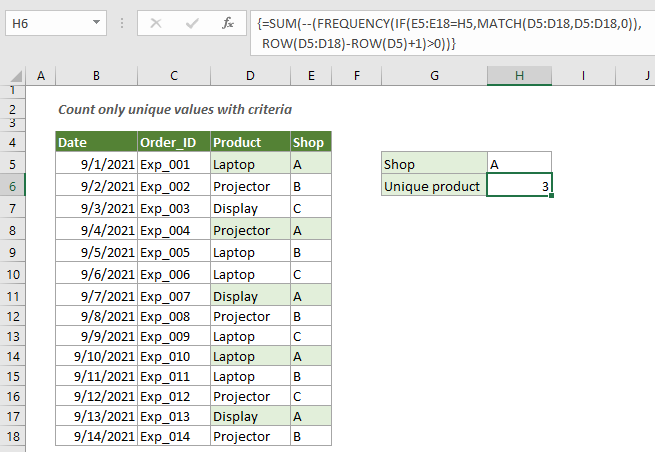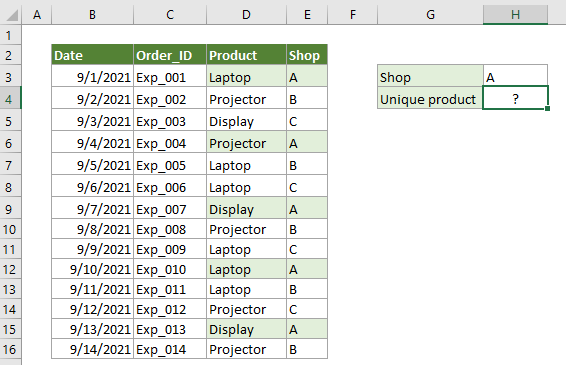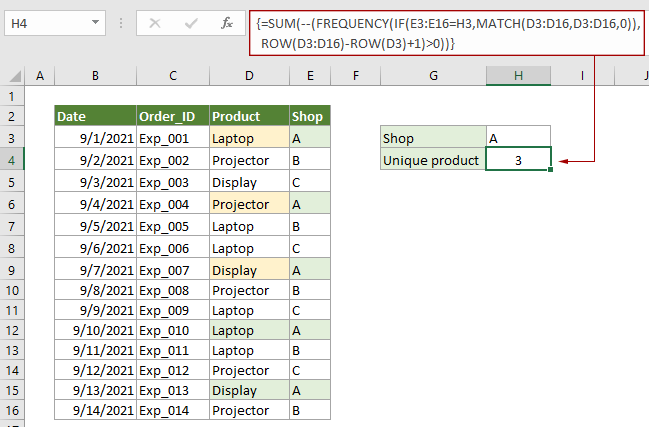## Count unique values with criteria in Excel

To count only unique values based on a specified criteria in another column, you can apply an array formula based on the SUM, FREQUENCY, MATCH and the ROW functions. This step-by-step guide helps you get through the most nerve-wracking usage of the formula.#### How to count unique values with criteria in Excel?

As the below product table shown, there are some duplicate products sold from the same shop in different dates, now, I want to get the unique count of the product which sold from the shop A, you can apply the below formula.Generic Formulas

{=SUM(--(FREQUENCY(IF(range=criteria,MATCH(vals,vals,0)),ROW(vals)-ROW(vals.firstcell)+1)>0))}

Arguments

Range: The range of cells contains the value that against the criteria;
Criteria: The criteria you want to count unique values based on;
Vals: The range of cells you want to count unique values from;
Vals.firstcell: The first cell of the range you want to count unique values from.

Note: This formula must be entered as an array formula. After applying the formula, if there are curly brackets wrapped around the formula, an array formula is successfully created.

How to use these formulas?

1. Select a blank cell to place the result.

2. Enter the below formula into it and then press the Ctrl + Shift + Enter keys simultaneously to get the result.

=SUM(--(FREQUENCY(IF(E3:E16=H3,MATCH(D3:D16,D3:D16,0)),ROW(D3:D16)-ROW(D3)+1)>0))Notes: In this formula, E3:E16 is the range contains the value that against the criteria, H3 contains the criteria, D3:D16 is the range contains the unique values you want to count, and D3 is the first cell of D3:D16. You can change them as you need.

How this formula works?

{=SUM(--(FREQUENCY(IF(E3:E16=H3,MATCH(D3:D16,D3:D16,0)),ROW(D3:D16)-ROW(D3)+1)>0))}

• IF(E3:E16=H3,MATCH(D3:D16,D3:D16,0)):
1) E3:E16=H3: Here check if value A existing in range E3:E16, and returns TRUE if it is found, returns FALSE if not. You will get an array like this {TRUE;FALSE;FALSE;TRUE;FALSE;FALSE;TRUE;FALSE;FALSE;TRUE;FALSE;}.
2) MATCH(D3:D16,D3:D16,0): The MATCH function gets the first location of each item in range D3:D16, and return an array like this {1;2;3;2;1;1;3;2;1;1;1;2;3;2}.
• IF({TRUE;FALSE;FALSE;TRUE;FALSE;FALSE;TRUE;FALSE;FALSE;TRUE;FALSE;},{1;2;3;2;1;1;3;2;1;1;1;2;3;2}): Now for each TRUE value in array 1, we will get the corresponding position in array 2, and for FALSE, we will get FALSE. Here you will get a new array as {1;FALSE;FALSE;2;FALSE;FALSE;3;FALSE;FALSE;1;FALSE;FALSE;3;FALSE}.
• ROW(D3:D16)-ROW(D3)+1: Here the ROW function returns the row number of reference D3:D16 and D3, and you will get {3;4;5;6;7;8;9;10;11;12;13;14;15;16}-{3}+1.
• Each number in the array subtracts number 3 then adds 1 and finally returns {1;2;3;4;5;6;7;8;9;10;11;12;13;14}.
• FREQUENCY({1;FALSE;FALSE;2;FALSE;FALSE;3;FALSE;FALSE;1;FALSE;FALSE;3;FALSE},{1;2;3;4;5;6;7;8;9;10;11;12;13;14}): Here the FREQUENCY function returns the frequency of each number in given array: {2;1;2;0;0;0;0;0;0;0;0;0;0;0}.
• =SUM(--({2;1;2;0;0;0;0;0;0;0;0;0;0;0}>0)):
1) {2;1;2;0;0;0;0;0;0;0;0;0;0;0}>0: Each number in the array compares with 0, and returns TRUE if greater than 0, otherwise, returns FALSE. And you will get a TRUE FALSE array like this {TRUE;TRUE;TRUE;FALSE;FALSE;FALSE;FALSE;FALSE;FALSE;FALSE;FALSE;FALSE;FALSE;FALSE};
2) --{TRUE;TRUE;TRUE;FALSE;FALSE;FALSE;FALSE;FALSE;FALSE;FALSE;FALSE;FALSE;FALSE;FALSE}: These two minus signs convert “TRUE” into 1 and “FALSE” into 0. Here you will get a new array as{1;1;1;0;0;0;0;0;0;0;0;0;0;0}.
3) SUM{1;1;1;0;0;0;0;0;0;0;0;0;0;0}: The SUM function sums all numbers in the array and returns the final result as 3.

#### Related functions

Excel SUM function
The Excel SUM function adds values

Excel FREQUENCY function
The Excel FREQUENCY function calculates how often values occur within a range of values, and then returns a vertical array of numbers.

Excel IF function
The Excel IF function performs a simple logical test which depending on the comparison result, and it returns one value if a result is TRUE, or another value if result is FALSE.

Excel MATCH function
The Excel MATCH function searches for a specific value in a range of cells, and returns the relative position of this value.

Excel ROW function
The Excel ROW function returns the row number of a reference.

#### Related formulas

Count Number Of Visible Rows In A Filtered List
This tutorial explains how to count number of visible rows in a filtered list in Excel with the SUBTOTAL function.

Count unique values in a range
This tutorial explains how to count only the unique values among duplicates in a list in Excel with specified formulas.

Count visible rows with criteria
This tutorial provides detailed steps to help you count visible rows with criteria.

Use COUNTIF on a non-contiguous range
This step-by-step guide demonstrates how to use the countif function on a non-contiguous range in Excel.

### The Best Office Productivity Tools

#### Kutools for Excel - Helps You To Stand Out From Crowd

 Popular Features: Find/Highlight/Identify Duplicates  |  Delete Blank Rows  |  Combine Columns or Cells without Losing Data  |  Round without Formula ... Super VLookup: Multiple Criteria  |  Multiple Value   |  Across Multi-Sheets  |  Fuzzy Lookup... Adv. Drop-down List: Easy Drop Down List  |  Dependent Drop Down List  |  Multi-select Drop Down List... Column Manager: Add a Specific Number of Columns  |  Move Columns  |  Unhide Columns  |  Compare Columns to Select Same & Different Cells ... Featured Features: Grid Focus  |  Design View  |  Big Formula Bar  |  Workbook & Sheet Manager  |  Resource Library (Auto Text)  |  Date Picker  |  Combine Worksheets  |  Encrypt/Decrypt Cells  |  Send Emails by List  |  Super Filter  |  Special Filter (filter bold/italic/strikethrough...) ... Top 15 Toolsets:  12 Text Tools (Add Text, Remove Characters ...)  |  50+ Chart Types (Gantt Chart ...)  |  40+ Practical Formulas (Calculate age based on birthday ...)  |  19 Insertion Tools (Insert QR Code, Insert Picture from Path ...)  |  12 Conversion Tools (Numbers to Words, Currency Conversion ...)  |  7 Merge & Split Tools (Advanced Combine Rows, Split Cells ...)  |  ... and more

Kutools for Excel Boasts Over 300 Features, Ensuring That What You Need is Just A Click Away...

Supports Office/Excel 2007-2021 and 365   |   Available in 44 Languages   |   Easy to Uninstall Completely#### Office Tab - Enable Tabbed Reading and Editing in Microsoft Office (include Excel)

• One second to switch between dozens of open documents!
• Reduce hundreds of mouse clicks for you every day, say goodbye to mouse hand.
• Increases your productivity by 50% when viewing and editing multiple documents.
• Brings Efficient Tabs to Office (include Excel), Just Like Chrome, Edge and Firefox.